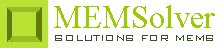SLIPFLO,len,wid,ht,slp,dP,vis,sel
Fluid flow in a wide rectangular channel with slip

len        length of the channel in µm
wid       width of the channel in µm
ht          height or depth of the channel in µm
slp        Navier slip length of the channel in nm
dP         applied pressure difference over the channel length in kPa
vis        dynamic viscosity in mPa-s
sel        number denoting the selected result.
Use 1 for hydraulic flow resistance, 2 for flow rate and 3 for average flow velocityNotes

The flow problems discussed under Mechanics > Fluidics > Microchannels > No-Slip, used the no slip boundary condition for solution. No slip boundary condition assumes that the velocity of the fluid particles in contact with the walls of the channel has the same velocity as the wall itself, which is equal to zero. But experimental studies of fluid flow through micro and nanochannels have shown that the point where the flow velocity drops to zero is a little beyond the channel walls. This length is called slip length or Navier length which is the distance from the wall where the tangent of the velocity field meets the normal axis. In no-slip flow calculations, this length is assumed to be zero. Flow studies have shown that this length ranges from 50nm to several micrometers depending on the type of fluid and flow conditions.

Pressure driven, steady state flow of an incompressible fluid through a straight microchannel is typically laminar in nature, where the viscous effects dominate and inertial effects can be neglected as characterized by very small Reynolds's number. Viscous effects leads to internal friction in the fluid and creates resistance to the flow of the fluid. Just like electrical resistance leads to Joule heating, hydraulic resistance leads to dissipation of kinetic energy into heat.

Understanding hydraulic resistance is vital to the design of a microchannel. Mathematically it is represented by the Hagen-Poiseuille law that relates constant pressure drop dP resulting in a constant fluid flow Q

dP = Rhyd Q

Hagen-Poiseuille law is analogous to the Ohm's law dV = RI that relates the current I through a wire of resistance R and an electrical potential drop of dV. Similar to electrical resistors connected in series, two channels with different hydraulic resistance can be modeled by summing the two hydraulic resistance together. On similar lines, two hydraulic resistors connected in parallel follows the law of additivity of inverse of their hydraulic resistances.

Use this design form to calculate the hydraulic resistance of a wide rectangular microfluidic channel with constant cross sectional area. If the channel has varying cross sectional area, the hydraulic resistance for individual channels with constant cross section can be found using this design form and then added together using the additivity law explained above. The flow rate can then be estimated using the Hagen-Poiseuille law. The average flow velocity is approximately one half of the maximum velocity which is at the center of the channel. To find the time the fluid takes to travel the full length of the channel, divide the length of the channel with the flow velocity.

The plot shows the velocity field for the Poiseuille flow in a wide rectangular channel mimicking two infinite plates with and without slip. The plot in blue color shows the velocity field along the center line parallel to the height of the channel with slip. The plot in red color shows the velocity field along the center line parallel to the height of the channel without slip. It shows that the flow with slip has a positive velocity at the channel walls. The maximum velocity can be found out from the velocity field plot which happens at the center of the channel.

Assumptions

-The Reynold's number is small Re << 1 and inertial effects can be neglected.
-The channels are long, rigid and narrow.
-The channel width is much larger than its height.
-The fluid has constant viscosity.
-Perfect hydrostatic pressure balance is assumed in the plane of the channel.